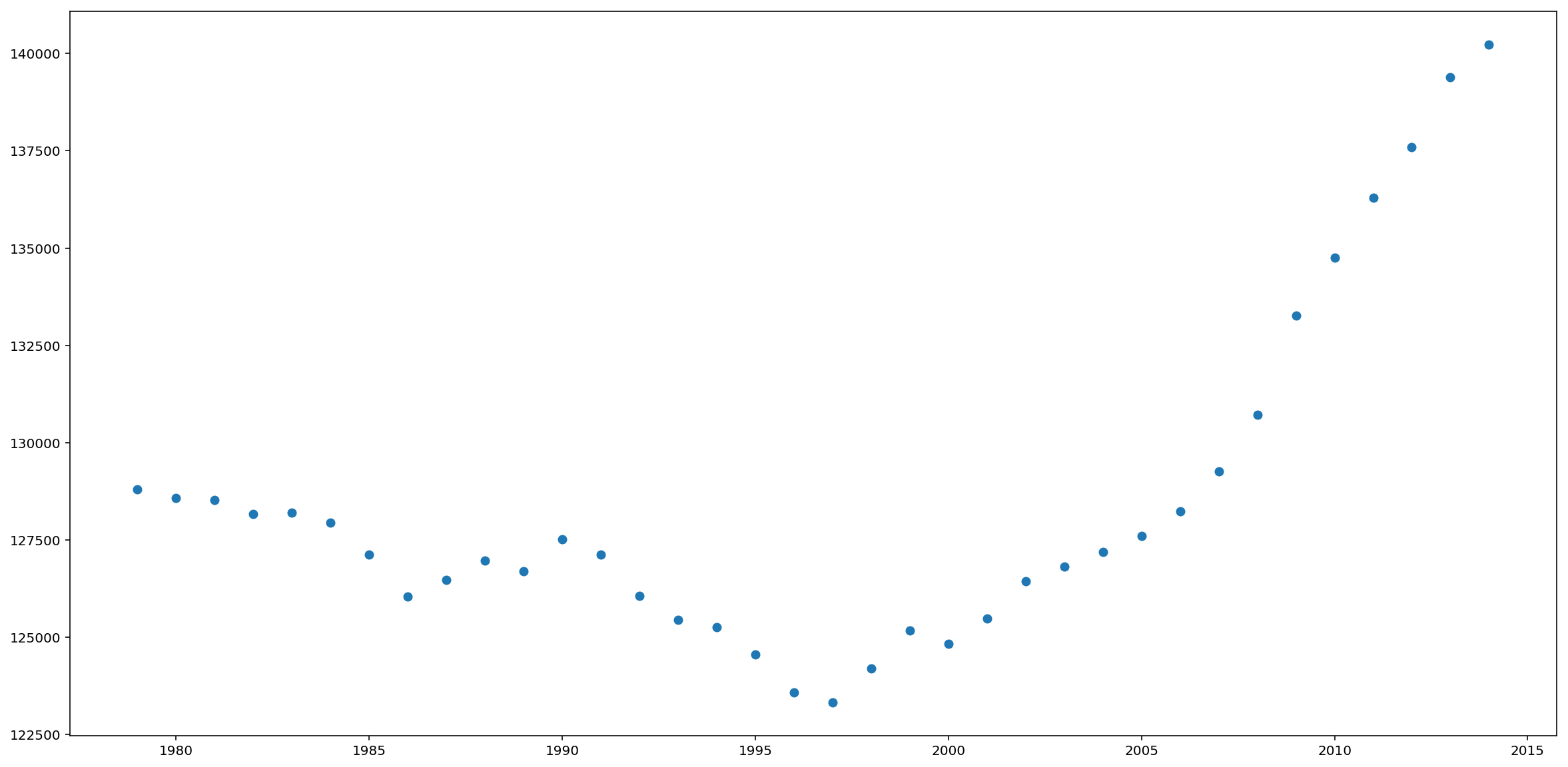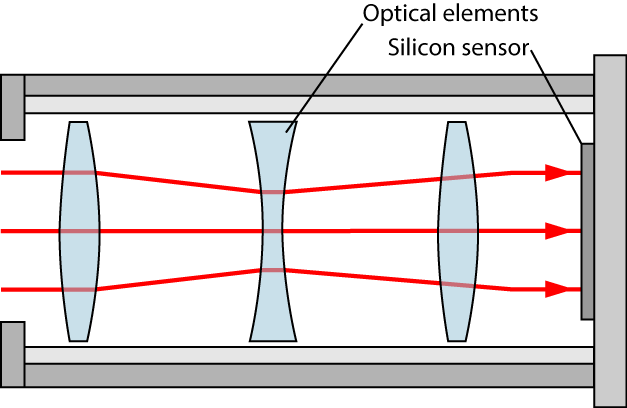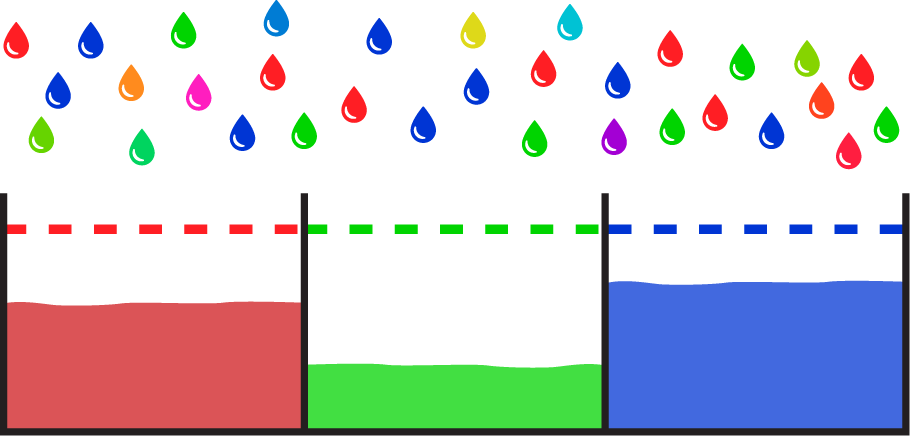RGL
Realistic Graphics Lab

# Homework 2¶

### CS328 — Numerical Methods for Visual Computing¶

Out on Monday 16.10, due on Monday 30.10.

This notebook contains literate code, i.e. brief fragments of Python surrounded by descriptive text. Please use the same format when submitting your answers. Begin your response to each problem with a  ## Solution   markdown cell. Since this exercise includes a number of supplementary discussions, questions are explicitly marked with a TODO marker.

Please keep in mind that homework assignments must be done individually.

## Problem -1: Warmup (not graded)¶

$$\newcommand{\vb}{\mathbf{b}} \newcommand{\vc}{\mathbf{c}} \newcommand{\vx}{\mathbf{x}} \newcommand{\mA}{\mathbf{A}} \newcommand{\mL}{\mathbf{L}} \newcommand{\mU}{\mathbf{U}} \newcommand{\mP}{\mathbf{P}} \newcommand{\mI}{\mathbf{I}}$$

The following Yes/No and paper & pencil questions are meant to check your comprehension of lecture and reading material in addition to linear algebra prerequisites.

1. Recall that a permutation matrix $\mP$ has exactly one entry with value $1$ in each row and column and zeros elsewhere. Prove that $\mP^{-1}=\mP^T$. You'll need the definitions of matrix multiplication and the matrix inverse, namely that $\mA\mA^{-1}=\mI$. (where $\mI$ is the identity matrix)

2. Which of the following parametric models can be fit to data using linear regression techniques? \begin{align*} f_1(x, \vc) &= \vc_0 + \vc_1 x^2 + \vc_2 \sin(x) - \sqrt{\vc_3}\\ f_2(x, \vc) &= \vc_0^3 x^3 - x\\ f_3(x, \vc) &= \exp(\vc_0 x)\\ \end{align*} here, $x$ represents the independent variable and $\vc$ is a vector containing the model parameters.

3. A matrix $\mA\in\mathbb{R}^{n\times n}$ is said to be symmetric and positive definite (SPD) iff $\mA=\mA^T$ and $\vx^T\mA\vx>0$ for all $\vx\in\mathbb{R}^n$ with $\vx\ne \mathbf{0}$. It's useful to know whether matrices are SPD, since specialized storage representations (don't store entries below the diagonal) and factorization algorithms (Cholesky decomposition) can exploit these properties, reducing the expense of linear system solving by approximately 50%.

Now recall the definition of the normal equations corresponding to the least squares system $\mA\vx\approx\vb$: $$\mA^T\mA\vx=\mA^T\vb.$$ Assuming that we'd like solve a least squares problem with this approach, it would naturally be useful to know whether the product matrix $\mA^T\mA$ is symmetric and positive definite so that these more efficient algorithms can be used. Can you prove that $\mA^T\mA$ is SPD? (you'll likely need to introduce some extra assumptions on $\mA$ for this)

## 0 Prelude¶

As in the last assignment we'll begin by importing essential NumPy/SciPy/Matplotlib components that are needed to complete the exercises. The first two lines instruct Matplotlib to embed figures directly into the notebook and render them in sufficient quality for modern high-DPI ("retina") displays.

In :
%matplotlib inline
%config InlineBackend.figure_format='retina'

import numpy as np
import scipy.linalg as la
from matplotlib import pyplot as plt


You will get to run numerical algorithms on high resolution images in this homework, which will involve a fair amount of plotting and visualization of intermediate results. The Matplotlib default settings unfortunately cause figures to be shown at a tiny resolution, so this next line changes the settings to make all figures large by default. The second line ensures low resolution images are rendered with sharp pixel contours instead of a blurry approximation, which will be helpful later on.

In :
plt.rcParams['figure.figsize'] = (20.0, 10.0)
plt.rcParams['image.interpolation'] = 'nearest'


# Problem 1: Fitting a function to data, a.k.a. Regression Analysis (15 points)¶

The following plot visualizes the total population of the Canton Vaud over a period of 35 years from 1979 to 2014.

In :
years = np.linspace(1979, 2014, 36)
population = np.array([128808, 128571, 128525, 128165, 128199, 127952, 127128, 126050, 126475, 126976,
126699, 127515, 127118, 126058, 125457, 125264, 124561, 123577, 123325, 124205,
125174, 124835, 125485, 126441, 126825, 127194, 127597, 128228, 129268, 130721,
133265, 134753, 136288, 137586, 139390, 140228], dtype=np.float64)
plt.plot(years, population, 'o');In this exercise, you will apply the tools of linear regression analysis and and linear least squares to fit an approximate parametric model to this dataset.

1. TODO: Solve a linear system via the LU factorization of the normal equations (via la.lu_factor() and la.lu_solve() similar to what was shown in class) to fit a degree-6 plynomial to this dataset, which maps from years to population values. Plot the original data, as well as your resulting polynomial (using a higher resolution with 300 evaluations between 1979 and 2014) in a figure below to compare the trend to the model.
Why are we using the normal equations here instead of directly applying the LU factorization?
Assuming this very simple model is correct, how large would you predict the population of Vaud to be in the year 2020?

2. TODO: Now create a similar figure where you fit again a degree-6 polynomial, but using the economy size QR factorization (and without the use of the normal equations). The functions to use are la.qr(matrix, mode='economic') and la.solve_triangular(). Which of the methods (1. or 2.) produces the solution with a lower residual?

3. TODO: What is the condition number of the product matrix $\mathbf{A}^T\mathbf{A}$ created by the normal equation approach in subproblem 1? (hint: use np.linalg.cond()). Relate this information to your observations in subproblem 2.

4. TODO: Use the same approch as in subproblem 1 (LU factorization), but this time without the normal equations (why?) to fit a degree-35 polynomial to the data. Does it result in a closer fit to the collected data? If so, explain why. Otherwise, what are the problems?

Hint: A degree-n polynomial has the form $p(x) = \sum_{k=0}^{n} c_k x^k$ with the $n+1$ constants $c_0, \dots, c_n$.

In :
# Solution part 1

In :
# Solution part 2

In :
# Solution part 3

In :
# Solution part 4


# 2 Developing a raw photograph (85 points)¶

Virtually any computer device in existence today is equipped with at least one digital camera; with over 5 billion users of digital photography, this technology has now fully permeated our society. In this exercise, you will get to build your own image processing pipeline that applies color space transformations to turn the raw data measured by a digital camera into a usable image that can be viewed on a computer screen. In the following, we will explain what this means, and how to implement the associated steps using tools from numerical linear algebra. But first, we shall begin with a brief review of the process that takes place when taking a picture.

A digital camera uses an assembly of optical elements to focus the incident light onto a silicon sensor that consists of millions of tiny regions arranged in a regular grid. The silicon is sensitive to light, and each small region on the sensor (generally referred to as a pixel) measures the portion of light that falls on it.When no picture is being taken, the sensor is either inactive or used for a live preview (as in mobile phones or smaller cameras). When the actual photograph is taken, it is important to be able to control the precise amount of time during which light is collected by the sensor. This is realized by means of the shutter, a small mechanical or electronic barrier that prevents light from reaching the sensor. The shutter can open and close very quickly, in about 1/4000th of a second.

When taking a picture, the camera performs a sequence of steps in rapid succession: first, the shutter opens, allowing light to reach the pixels on the sensor. For a brief duration (known as the exposure time), the sensor collects all light that reaches the surface. To be able to distinguish colors, each pixel on the sensor is covered with a tiny filter that will only permit certain wavelengths of light to pass through.Due its physical nature, light reaches the sensor in discrete amounts not unlike droplets of rain that fill a number of buckets with water. When the exposure time is very short, each pixel may only have received a few droplets, and the image is very noisy. When the exposure time is too long, the buckets will fill up completely, and it is impossible to recover a usable image. Once the exposure time has elapsed, the shutter closes so that the measurement ceases to change any further.

Once the measurement is frozen, the camera proceeds to perform a full readout of the data associated with each pixel (i.e. the "fill height" of each bucket in the illustration above). This data is known as a raw image: a representation of the original sensor data without any additional processing. Raw images play a similar role as negatives in traditional photography: they contain all of the information that is needed to eventually create an image, but they aren't yet usable as an image on their own. Converting a raw image into an actual image entails decoding the information on the sensor and translating this data into displayable red/green/blue intensities that "make sense" on a computer screen, and which reproduce the colors that a human would have observed in the moment when the photo was taken.

Analogous to classical photography, the process of turning the raw sensor data into a usable image is referred to as "developing" the image. Cell phone cameras generally perform this step automatically without user intervention. On the other hand, most professional cameras allow the user to choose between developing the image automatically within the camera or storing undeveloped raw images that can be manually developed by the user later on. The latter has become extremely popular, since raw image contain more information than would be available in a fully processed image (such as a JPEG image). For instance, JPEG files may not be able to represent the full range of colors perceived by the camera's sensor, and the lossy compression implies that there is some loss of image quality. A range of commercial (Adobe Photoshop, Lightroom, ..) and free (RawTherapee, darktable, ..) software tools can be used to develop raw image with full artistic control over the output.

Developing an image can be an arbitrarily complex process, though the simplest version can be reduced to only four steps:

2. Demosaicing
3. Color space transformation
4. Gamma correction

We will now walk through each of these steps (slightly out of order):

Begin by downloading the following file (~72 megabytes), which contains the raw sensor data of two photographs taken with a Canon 6D camera: http://rgl.s3.eu-central-1.amazonaws.com/media/uploads/wjakob/2016/10/20/lacleman_raw.npz.

Place the file into the same directory as the CS328 - Homework 2.ipynb file so that it is easy to load from Python. The following set of commands then import the file and extract all relevant data (this will take a few seconds):

In :
data = np.load("lacleman_raw.npz")
tree = data['tree']
whitebal = data['whitebal']
patches_target = data['patches_target']


The file contains three 2D NumPy arrays—two high resolution photographs (tree and whitebal) with approximately 20 million pixels each, and a mysterious small array (patches_target) that we'll need later on. We will only focus on the tree array for now.

In :
print(tree.shape)
print(whitebal.shape)
print(patches_target.shape)

(3666, 5494)
(3666, 5494)
(24, 3)


## 2.2 Demosaicing (15 points)¶

Let's try to display one of the raw images using Matplotlib!

If you get a IOPub data rate exceeded error message here, please restart your jupyter notebook with the appropriate command-line flag and a sufficiently high limit, e.g:
jupyter notebook --NotebookApp.iopub_data_rate_limit=10000000000
In :
plt.imshow(tree);